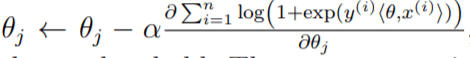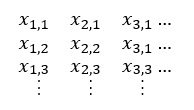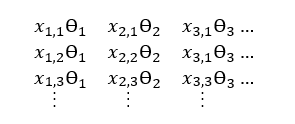Want to share your content on R-bloggers? click here if you have a blog, or here if you don't.In a previous post, we showed how using vectorization in R can vastly speed up fuzzy matching. Here, we will show you how to use R’s vectorization functionality to efficiently build a logistic regression model. Now we could just use the caret or stats packages to create a model, but building algorithms from scratch is a great way to develop a better understanding of how they work under the hood.

In writing the logistic regression algorithm from scratch, we will consider the following definitions and assumptions:

x = A dxn matrix of d predictor variables, where each column xi represents the vector of predictors corresponding to one data point (with n such columns i.e. n data points)

d = The number of predictor variables (i.e. the number of dimensions)

n = The number of data points

y = A vector of labels i.e. yi equals the label associated with xi; in our case we’ll assume y = 1 or -1

Θ = The vector of coefficients, Θ1, Θ2…Θd trained via gradient descent. These correspond to x1, x2…xd

α = Step size, controls the rate of gradient descent

Logistic regression, being a binary classification algorithm, outputs a probability between 0 and 1 of a given data point being associated with a positive label. This probability is given by the equation below:Recall that <Θ, x> refers to the dot product of Θ and x.

In order to calculate the above formula, we need to know the value of Θ. Logistic regression uses a method called gradient descent to learn the value of Θ. There are a few variations of gradient descent, but the variation we will use here is based upon the following update formula for Θj:This formula updates the jth element of the Θ vector. Logistic regression models run this gradient descent update of Θ until either 1) a maximum number of iterations has been reached or 2) the difference between the current update of Θ and the previous value is below a set threshold. To run this update of theta, we’re going to write the following function, which we’ll break down further along in the post. This function will update the entire Θ vector in one function call i.e. all j elements of Θ.

```
# function to update theta via gradient descent
update_theta <- function(theta_arg,n,x,y,d,alpha)
{

# calculate numerator of the derivative
numerator <- t(replicate(d , y)) * x

# perform element-wise multiplication between theta and x,
# prior to getting their dot product
theta_x_prod <- t(replicate(n,theta_arg)) * t(x)
dotprod <- rowSums(theta_x_prod)

denominator <- 1 + exp(-y * dotprod)

# cast the denominator as a matrix
denominator <- t(replicate(d,denominator))

# final step, get new theta result based off update formula
theta_arg <- theta_arg - alpha * rowSums(numerator / denominator)

return(theta_arg)

}

```

## Simplifying the update formula

To simplify the update formula for Θj, we need to calculate the derivative in the formula above.

Let’s suppose z = (yi)(<Θ, xi>). We’ll abbreviate the summation of 1 to n by simply using Σ.

Then, calculating the derivative gives us the following:

Σ (1 / exp(1 + z)) * exp(z) * xiyi

= Σ (exp(z) / exp(1 + z)) * xiyi

Since exp(z) / (1 + exp(z)) is a known identity for 1 / (1 + exp(-z)), we can substitute above to get:

Σ 1 / (1 + exp(-z)) * xiyi

= Σ xiyi / (1 + exp(-z))

Now, substituting (yi)(<Θ, xi>) back for z:

= Σ xiyi / (1 + exp(-(yi)(<Θ, xi>)))

Plugging this derivative result into the rest of the update formula, the below expression tells us how to update Θj:

Θj ← Θj – αΣ xiyi / (1 + exp(-(yi)(<Θ, xi>)))

To convert this math into R code, we’ll split up the formula above into three main steps:

• Calculate the numerator: xiyi
• Calculate the denominator: (1 + exp(-(yi)(<Θ, xi>)))
• Plug the results from first two steps back into the formula above

• The idea to keep in mind throughout this post is that we’re not going to use a for loop to update each jth element of Θ. Instead, we’re going to use vectorization to update the entire Θ vector at once via element-wise matrix multiplication. This will vastly speed up the gradient descent implementation.

## Step 1) Calculating the numerator

In the numerator of the derivative, we have the summation of 1 to n of yi times xi. Effectively, this means we have to calculate the following:

y1x1 + y2x2…+ynxn

This calculation needs to be done for all j elements in Θ to fully update the vector. So, we actually need to run the following calculations:

y1x1,1 + y2x1,2…+ynx1,n

y1x2,1 + y2x2,2…+ynx2,n

y1xd,1 + y2xd,2…+ynxd,n

Since y is a vector, or put another way, is an nx1 matrix, and x is a dxn matrix, where d is the number of predictor variables i.e. x1, x2, x3…xd, and n is the number of labels (how many predicted values we have), then we can compute the above calculations by creating a dxn matrix where each row is a duplicate of y. This way we have d duplicates of y. Each ith element of x (i.e. xi) corresponds to the ith column of x. So xj,i refers to the element in the jth row and ith column of x.In the above expression, instead of doing traditional matrix multiplication, we are going to do element-wise multiplication i.e. the jth, ith element of the resultant matrix will equal the jth, ith elements of each matrix multiplied by each other. This can be done in one line of R code, like below:

```
# calculate numerator of the derivative of the loss function
numerator <- t(replicate(d , y)) * x

```

Initially we create a matrix where each column is equal to y1, y2,…yn. This is replicate(d, y). We then transpose this so that we can perform the element-wise multiplication described above. This element-wise multiplication gets us the following dxn matrix result:Notice how the sum of each jth row corresponds to the each calculation above i.e. the sum of row 1 is Σxiyi for j = 1, the sum of row 2 is Σxiyi for j = 2…the sum of row d is Σxiyi for j = d. Rather then using slower for loops, this allows us to calculate the numerator of the derivative given above for each element (Θj) in Θ using vectorization. We’ll postpone doing the summation until after we’ve calculated the denominator piece of the derivative.

## Step 2) Calculating the denominator

To evaluate the denominator of our formula, we need to first calculate the dot product of Θ and x. We do this similarly to our numerator calculation:

```
theta_x_prod <- t(replicate(n,theta_arg)) * t(x)
dotprod <- rowSums(theta_x_prod)

```

The above code corresponds to the math below:

t(replicate(n,theta_arg))t(x)Thus, theta_x_prod equals:The sum of each ith row is, by definition, the dot product of Θ and xi. Thus, by calculating the sum of each row, we can get a vector like this:

<Θ, x1>, <Θ, x2>, <Θ, x3>, … <Θ, xn>

The calculation above is handled in R by the rowSums(theta_x_prod) code above.

Next, we plug the dotprod result into our formula to calculate the denominator. We then use the familiar transpose / replicate idea to create a matrix where each jth row will be used in updating Θj.

```
denominator <- 1 + exp(-y * dotprod)

# cast the denominator as a matrix
denominator <- t(replicate(d,denominator))

```

## Step 3) Finishing the update formula

The last line of code in the function for updating theta is finishing the rest of the formula:

```
# final step, get new theta result based off update formula
theta_arg <- theta_arg - alpha * rowSums(numerator / denominator)

```

## Finally…putting it all together

The next function we need will essentially call our update_theta function above until either the change in the updated versus previous theta value is below a threshold, or a maximum number of iterations has been reached.

```

# wrapper around update_theta to iteratively update theta until the maximum number of iterations is reached
get_final_theta <- function(theta,x,y,threshold,alpha=0.9,max_iter = 100)
{

n <- ncol(x)
d <- length(theta)

first_iteration <- TRUE
total_iterations <- 0

# while the number of iterations is below the input max allowable,
# update theta via gradient descent
new_theta <- theta
while(total_iterations <= max_iter)
{

first_iteration <- FALSE

# create copy of theta for comparison between new and old
old_theta <- new_theta
new_theta <- update_theta(new_theta,n,x,y,d,alpha)

diff_theta <- sqrt(sum((new_theta - old_theta)**2))

if(diff_theta < threshold) {break}

# index the iteration number
total_iterations <- total_iterations + 1

}

# return the train theta parameter
return(new_theta)

}

```

Lastly, we write a simple function to calculate the predicted probability from the logistic regression model given a final theta vector:```
# wrapper around the gradient descent algorithm implemented in the two functions above
# ~ outputs logistic regression results
lr <- function(x, y, theta_arg)
{
result <- sapply(1:ncol(x_arg) , function(index) 1 / (1 + exp(sum(theta_arg * x_arg[,index]))))
return(result)

}

```

Now, if we want to use our code to train a logistic regression model, we can do it like below, using a randomized dataset as an example.

```
# create initial values for theta
theta <- rep(0.5, 100)

# initialize other needed parameters
alpha <- 0.9
threshold <- 1
max_iter <- 100

# generate random matrix of predictors
train_x <- lapply(1:100, function(elt) sample(1:10000, 100, replace = TRUE))
train_x <- Reduce(cbind, x)

# set seed for reproducibility
set.seed(2000)

# generate random labels
train_y <- sample(c(1, -1), 100, replace = TRUE)

# get trained theta
train_theta_result <- get_final_theta(theta, train_x , y, 1, .9, max_iterations = 100)

# run trained model on dataset
train_result_predictions <- lr(train_x, train_theta_result)

```

Then, if we want to use the trained logistic regression model on a new test data, we can use the train_theta_result vector above.

```
# set seed for reproducibility
set.seed(1234)

# generate randomized test dataset
test_x <- lapply(1:100, function(elt) sample(1:10000, 100, replace = TRUE))
test_x <- Reduce(cbind, x)

# get predictions on test dataset
test_result_predictions <- lr(test_x, train_theta_result)

```

That’s it for this post! Please check out other R posts here: http://theautomatic.net/category/r/

The post How to build a logistic regression model from scratch in R appeared first on Open Source Automation.